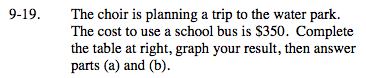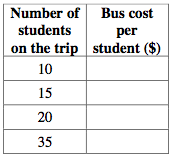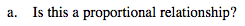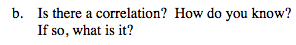### Home > MC2 > Chapter 9 > Lesson 9.1.2 > Problem9-19

9-19.For each number in the table, divide \$350 by the number of students to find how much the bus will cost for each student going on the trip.Does the cost for each student stay the same as the number of students increase?

No, as the number of students increase, the cost of the bus per student decreases.
Therefore, this is not a proportional relationship.Describe the characteristics of the graph of these data points.

Complete the table in the eTool below to graph the results.
Click the link at right for the full version of the eTool: MC2 9-19 HW eTool